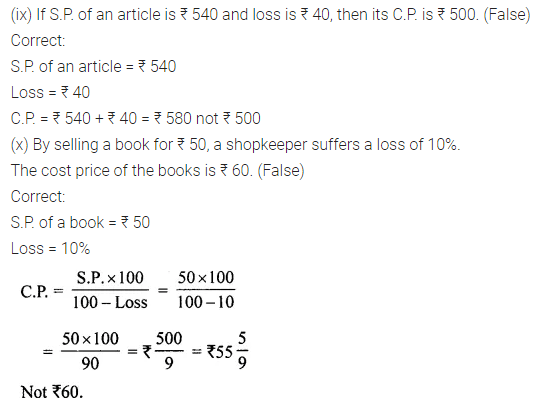# ML Aggarwal Class 7 Solutions for ICSE Maths Chapter 7 Percentage and Its Applications Objective Type Questions

## ML Aggarwal Class 7 Solutions for ICSE Maths Chapter 7 Percentage and Its Applications Objective Type Questions

Question 1.
Fill in the blanks:
(i) 6% of ₹ 50 = ……….
(ii) If 25% of a number is 12, then the number is ……….
(iii) The mixed fraction 1$$\frac { 3 }{ 4 }$$ converted to percentage form is
(iv) If a number increases from 20 to 28, then the increasing percentage is ………
(v) If cost price is ₹ 400 and loss is 15%, then the selling price is ……..
(vi) The profit or loss percentage is always calculated on ……….
(vii) The simple interest on a sum of ₹ 5600 at 8% p.a. for one year is ……….
(viii) 135% converted to decimal is ………
(ix) ……… is 50% more than 60.
(x) 25 mL is ………… percent of 5 litres.
Solution:Question 2.
State whether the following statements are true (T) or false (F):
(i) 20% more than 30 is 36.
(ii) The ratio 2 : 5 converted to percentage is 60%.
(iii) 6$$\frac { 1 }{ 4 }$$ % expressed as a fraction is $$\frac { 1 }{ 16 }$$.
(iv) 80% of 450 m is equal to 360 m.
(v) If a number decreases from 20 to 15, then the decrease is 25%.
(vi) If Feroz obtains 336 marks out of600 marks, then the percentage of marks obtained by him is 33.6.
(vii) 0.018 is equivalent to 8%.
(viii) 250 cm is 4% of 1 km.
(ix) If S.P. of an article is ₹ 540 and loss is ₹ 40, then its C.P. is ₹ 500.
(x) By selling a book for ₹ 50, a shopkeeper suffers a loss of 10%. The cost price of the books is ₹ 60.
Solution:Multiple Choice Questions

Choose the correct answer from the given four options (3 to 16):
Question 3.
The ratio 2 : 3 expressed as percent is
(a) 40%
(b) 60%
(c) 66$$\frac { 2 }{ 3 }$$ %
(d) 33$$\frac { 1 }{ 3 }$$ %
Solution:Question 4.
The ratio of Fatima’s income to her saving is 4 : 1. The percentage of money saved by her is
(a) 20%
(b) 25%
(c) 40%
(d) 80%
Solution:Question 5.
225% is equal to
(a) 2 : 3
(b) 3 : 2
(c) 4 : 9
(d) 9 : 4
Solution:Question 6.
If 30% of x is 72, then x is equal to
(a) 120
(b) 240
(c) 360
(d) 480
Solution:Question 7.
If x% of 80 = 12, then x is equal to
(a) 15
(b) 20
(c) 25
(d) 30
Solution:Question 8.
0.025 when expressed as a percent is
(a) 250%
(b) 25%
(c) 4%
(d) 2.5%
Solution:Question 9.
In class, 45% of students are girls. If there are 22 boys in the class, then the total number of students in the class is
(a) 30
(b) 36
(c) 40
(d) 44
Solution:Question 10.
What percent of $$\frac { 1 }{ 7 }$$ is $$\frac { 2 }{ 35 }$$ ?
(a) 20%
(b) 25%
(c) 30%
(d) 40%
Solution:Question 11.
If a man buys an article for ₹ 80 and sells it for ₹ 100, then gain percentage is
(a) 20%
(b) 25%
(c) 40%
(d) 125%
Solution:Question 12.
If a man buys an article for ₹ 120 and sells it for ₹ 100, then his loss percentage is
(a) 10%
(b) 20%
(c) 25%
(d) 16$$\frac { 2 }{ 3 }$$%
Solution:Question 13.
The salary of a man is ₹ 24000 per month. If he gets an increase of 25% in the salary, then the new salary per month is
(a) ₹ 2500
(b) ₹ 28000
(c) ₹ 30000
(d) ₹ 36000
Solution:Question 14.
On selling an article for ₹ 100, Renu gains ₹ 20. Her gain percentage is
(a) 25%
(b) 20%
(c) 15%
(d) 40%
Solution:Question 15.
The simple interest on ₹ 6000 at 8% p.a. for one year is
(a) ₹ 600
(b) ₹ 480
(c) ₹ 400
(d) ₹ 240
Solution:Question 16.
If Rohit borrows ₹ 4800 at 5% p.a. simple interest, then the amount he has to return at the end of 2 years is
(a) ₹ 480
(b) ₹ 5040
(c) ₹ 5280
(d) ₹ 5600
Solution:Value Based Questions

Question 1.
One bad apple is accidentally mixed with some good apples in a basket. As a result of which 25% of the total apples go bad. Now the number of good apples in the basket is 30. Find the number of good apples kept in the basket previously. What will happen if one bad person is mixed with some good ones?
Solution:Question 2.
There is a group of 50 people who are patriotic out of which 40% believe in non-violence. Find the number of persons who believe in non-violence. Explain the importance of non-violence in patriotism.
Solution:Higher Order Thinking Skills (HOTS)

Question 1.
A person preparing medicine wants to convert 15% alcohol solution into 32% alcohol solution. Find how much pure alcohol should he mix with 400 mL of 15% alcohol solution to obtain it.
Solution:Question 2.
A manufacturer sells an item to an agency at a profit of 25%. The agency sells the item to a shopkeeper at 10% profit and shopkeeper sells the item at a profit of 20%. If the selling price of the item is ₹ 594, find the manufacturing price.
Solution: